Courses

# Test: Traffic Engineering - 2

## 20 Questions MCQ Test Topicwise Question Bank for Civil Engineering | Test: Traffic Engineering - 2

Description
This mock test of Test: Traffic Engineering - 2 for Civil Engineering (CE) helps you for every Civil Engineering (CE) entrance exam. This contains 20 Multiple Choice Questions for Civil Engineering (CE) Test: Traffic Engineering - 2 (mcq) to study with solutions a complete question bank. The solved questions answers in this Test: Traffic Engineering - 2 quiz give you a good mix of easy questions and tough questions. Civil Engineering (CE) students definitely take this Test: Traffic Engineering - 2 exercise for a better result in the exam. You can find other Test: Traffic Engineering - 2 extra questions, long questions & short questions for Civil Engineering (CE) on EduRev as well by searching above.
QUESTION: 1

### Which one of the following diagrams illustrates the relation between speed 'u' and density 'k' of traffic flow?

Solution:

At u = 0,
k = kmax
u = umax k → 0
The curve (a) satisfies these conditions.
Curve (c) represents relation between density and volume of traffic.

QUESTION: 2

### Which one of the following methods of O-D traffic surveys is conducted for comprehensive analysis of traffic and transportation data?

Solution:

Comprehensive analysis of traffic and transportation data require:
- Origin and destination in each zone
- Mode of transportation
- Number of vehicle and passengers in each vehicle
- Purpose of each trip
- Selection of route
- Length of trip
- Intermediate stops and their reason etc.
All this can be collected by Road side interview method.

QUESTION: 3

### The lost time due to starting delay on a traffic signal is noted to be 3s, the actual green time is 25s and yellow time is 3s. How much is the effective green time?

Solution:

Effective green time = Actual green time + Yellow time - lost time
= 25 + 3 - 3
= 25 seconds

QUESTION: 4

In speed and delay study, if the average journey time on a stretch of road length of 3.5 km is 7.55 minutes and the average stopped delay is 1.8 minutes, the average running speed will be, nearly

Solution:

Average running time = Average journey time - Average stopped delay
= 7.55 - 1.8
= 5.75 minutes
Average running speed =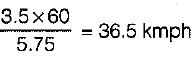QUESTION: 5

If L is the length of vehicle in meters, C is the clear distance between two consecutive vehicles (Stopping sight distance), V is the speed of vehicles in km/hour; then the maximum number (N) of vehicles/hour is equal to

Solution:

Maximum number of vehicles per hour =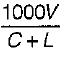QUESTION: 6

When the speed of the traffic flow becomes zero, then

Solution:

At zero speed density is maximum and volume is zero.

QUESTION: 7

It was noted that on a section of road, the free speed was 80 kmph and the jam density was 70 vpkm. The maximum flow in vph that could be expected on this road is

Solution: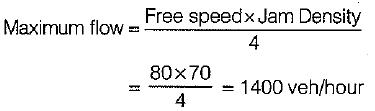QUESTION: 8

If the normal flows on two approach roads at an intersection are respectively 500 pcu per hr and 300 pcu per hr, the saturation flows are 1600 pcu per hr on each road and the total lost time per signal cycle is 16 s, then the optimum cycle time by Webster’s method is

Solution:

Optimum cycle time,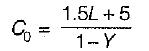L = Total lost time per cycle = 16 sec
Y = y1 + y2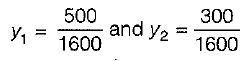∴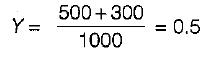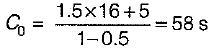QUESTION: 9

When two roads with two-lane, two-way traffic, cross at an uncontrolled intersection, the total number of potential major conflict points would be

Solution:

Point of potential conflicts depend on the number of lanes on intersecting lanes.
For two way traffic on a right angled road intersection, the conflict points are 24 whereas for two way traffic on T-intersection the conflict point are 18 only.

QUESTION: 10

An Enoscope is used for measuring

Solution:
QUESTION: 11

Matching List-I (Traffic flow characteristics) with Llst-ll (Figure/symbol) and select the correct answer using the codes given below the lists: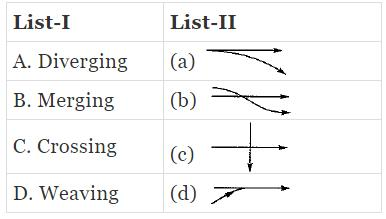Codes: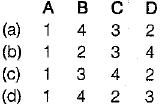Solution:
QUESTION: 12

The traffic conflicts that may occur in a rotary intersection are

Solution:
QUESTION: 13

In which of the following traffic signal system are the cycle length and cycle division are automatically varied?

Solution:

Flexible progressive system (most efficient method of signalling).

QUESTION: 14

Matching List-I with List-Il and select the correct answer using the codes given below the lists: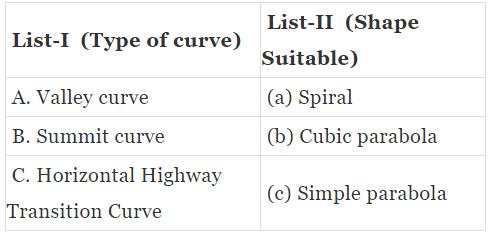Codes: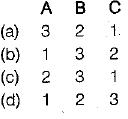Solution:
QUESTION: 15

Traffic volume is equal to

Solution:
QUESTION: 16

With increase in speed of the traffic stream, the maximum capacity of the lane

Solution:
QUESTION: 17

When the speed of traffic flow becomes zero, then

Solution:
QUESTION: 18

The most efficient traffic signal system is

Solution:
QUESTION: 19

A traffic rotary is justified where

Solution:
QUESTION: 20

When a number of roads are meeting at a point and only one of the roads is important, then the suitable shape of rotary is

Solution: